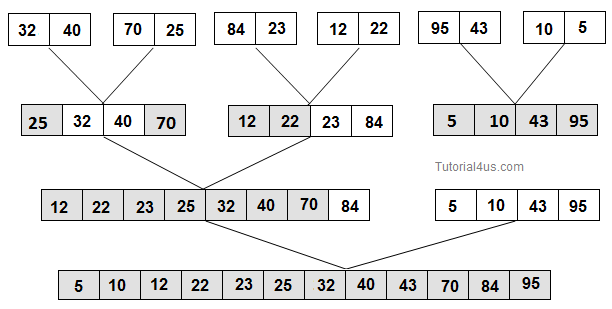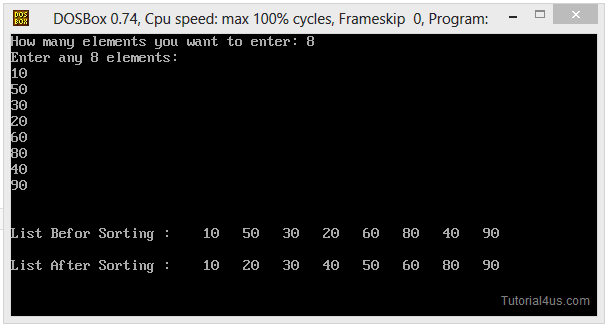# Merge Sort in C++

## Merge Sort in C++

Merge Sort is a sorting technique which divides the array into sub-arrays which have size 2 and merge (combined) adjacent (near) pair. After first process you have n/2 array of size 2. this process is repeated until there is only one array remaining of size n.## Merge Sort Program in C++

```
#include<iostream.h>
#include<conio.h>

void mergesort(int a[],int n)
{
int b,c,low1,high1,high2,low2;
int i,k,j;
c=1;
while(c<n)
{
low1=0;
k=0;
while(low1+c<n)
{
low2=low1+c ;
high1=low2-1;
if(low2+c-1<n)
high2=low2+c-1;
else
high2=n-1;
i=low1;
j=low2;
while(i<=high1 && j<=high2)
{
if(a[i]<=a[j])
b[k++]=a[i++];
else
b[k++]=a[j++];

}
while(i<=high1)
b[k++]=a[i++];
while(j<=high2)
b[k++]=a[j++];
low1=high2+1;
}
i=low1;
while(k<n)
b[k++]=a[i++];
for(i=0; i<n; i++)
a[i]=b[i];
c=c*2;
}
}

main()
{
int a,i,n;
clrscr();

cout<<("How many elements you want to enter: ";
cin>>n;
cout<<"Enter any<<n<<"Elements: \n";
for(i=0; i<n; i++)
{
cin>>a[i];
}

cout<<"\n\nList Befor Sorting : ";
for(i=0; i<n; i++)
{
delay(400);
cout<<a[i];
}
mergesort(a,n);

cout<<"\n\nList After Sorting : ";
for(i=0; i<n; i++)
{
delay(400);
cout<<a[i];
}
getch();
return 0;
}
```